•Fire Opal
• Topics
• Benchmarking results

# Performance improvement with Fire Opal

Evaluating Fire Opal performance improvement across multiple benchmark algorithms

## Introduction

Benchmarks are a common industry practice to determine the performance of quantum computing systems. The usefulness of a quantum computer is ultimately determined by its ability to successfully implement meaningful and relevant quantum algorithms in reasonable times and with reasonable resources. Benchmark measurements are especially important in the Noisy Intermediate-Scale Quantum (NISQ) era as a means of evaluating the scalability and feasibility of using available hardware for specific use cases.

Fire Opal boosts algorithmic success on quantum computers, and makes it possible to run algorithms on NISQ devices. This article provides an overview of the benchmarked improvements of Fire Opal run on IBM backends, compared to bare execution without Fire Opal. These benchmarks can help to set expectations for what sorts of improvements you will see with Fire Opal, but it is still highly encouraged to try it out yourself as our error suppression pipeline is constantly improving.

Benchmarks were carried out across a set of algorithms with widely varying characteristics (connectivity, circuit depth, etc): Bernstein–Vazirani (BV), quantum Fourier transform (QFT), Grover's search, quantum approximate optimization algorithm (QAOA), variational quantum eigensolver (VQE), and quantum error correction (QEC). Certain algorithms are inherently better suited for certain devices, due to device connectivity and layouts, but Fire Opal generally boosts algorithmic success probability across devices and algorithms.

For a detailed look at our methodologies and results, you can reference our technical manuscript.

## Configuration information

The results were computed on IBM devices Jakarta, Lagos, and Guadalupe. Jakarta and Lagos are both 7-qubit devices, while Guadalupe is a 16 qubit device.

The benchmarks were compiled using the following conditions:

ComponentDefaultFire Opal
Compilation MethodQiskit level 3 Sabre compiler, best-out-of-four seeds.Fire Opal Compilation passes and layout-selection optimization.
Quantum Logic GatesDefaultFire Opal autonomosly optimized two-qubit gates.
Error Mitigation strategiesNoneFire Opal optimal context-aware embedding of crosstalk-supressing dynamical decoupling sequences and Q-CTRL scalable measurement-error mitigation.

The default benchmark uses the default settings provided by IBM alongside the most aggressive compilation scheme the platform provides.

## Algorithms and Results Summary

The table below provides a quick overview of each of the algorithms used for benchmarking and how performance was evaluated. As expected output varies across algorithms, the method for comparing similarity to the "right" answer also differs. The observed performance enhancement is summarized for the purpose of quick comparison. Explanations in greater detail can be found below the table.

AlgorithmCharacteristicsEvaluation MetricPerformance enhancement
Bernstein–Vazirani (BV)Shallow and Sequential - circuit depth scales linearly with circuit width (for full connectivity) and native entangling gates do not overlap.Success probability of returning the all ‘1’ state. This selection constitutes a worst-case scenario using the most challenging (state-dependent) oracle.> 1000× improvement in success probability, up to 16 qubits.
Quantum Fourier Transform (QFT)Intermediate depth and partially sequential - circuit depth scales quadratically with circuit width (for full connectivity). Parts of the algorithm can be parallelized.Success probability averaged over 16-32 initial states (chosen to be inverse QFT of single bitstrings).7× (3×) over default (expert), up to 7 qubits.
Grover SearchHigh depth, scaling depends on the implementation of the multi-CX gate. The depth can be reduced by allowing the addition of ancilla qubits.Selectivity and distance (circuit infidelity) to the ideal target probability distribution over 32 different 5Q target states.8× improvement in circuit fidelity; transform selectivity (S < 0 → S > 1) for all target states, up to 5 qubits.
Quantum Approximate Optimization AlgorithmDepth and density depend on the specific problem. Problem under test is a five qubit max-cut type Hamiltonian with depth and density similar to the QFT class.Structural similarity metric between the ideal and measured cost landscapes.28× improvement in the Structural similarity metric.
Variational Quantum EigensolverDepth and density depend on the specific ansatz. Problem under test uses four- and six-qubit $R_y$ ansatzes with linear entaglement. Each round of ansatz has depth and density in the BV class.Ground state energy accuracy for BeH2 molecule and deviation from the ideal set of Pauli expectation values using the Pearson distance.5× error reduction in the mean ground state energy prediction, and 16× improvement in the Pearson distance of the measured Pauli expectation values.
Quantum Error Correction codesDepth in the QFT class. Due to device topology, the encoding block and each of syndrome inference blocks are highly sequential.Agreement between syndrome readout and errors inferred from direct data qubits readout.4× improved error detection in the repetition code and 3.3× for the full five qubit code (total 9 qubits).
Quantum VolumeIntermediate depth (similar to QFT) with maximal density. Operations are maximally parallelized.Mean heavy output (HO) Probability, averaged over 300 random circuits sampled with 1000 shots each.QV increased from 32 to 64.

## Detailed results per algorithm

### Bernstein–Vazirani (BV)

An $N$-qubit BV algorithm has a deterministic answer in the form of a single $(N − 1)$-qubits bitstring. The answer or target bitstring is determined by the choice of oracle circuit. The oracle circuit is chosen so that the answer is given by the all "1" bitstring. This oracle is the most challenging one among the possible BV oracles because its circuit includes the highest number of entangling gates. Therefore, the results shown here can be seen as the worst performance among all possible oracles. The circuit depth here would scale linearly with circuit width if the physical device had full connectivity and native entangling gates did not overlap.

In the following figure, the success probability of a BV algorithm is shown as a function of the number of qubits. Default and Fire Opal settings are described in the configuration table in the previous section. Associating the mode of the distribution with the correct answer, Fire Opal returns the correct answer for all system sizes while the default method fails to return the correct answer beyond 8 qubits.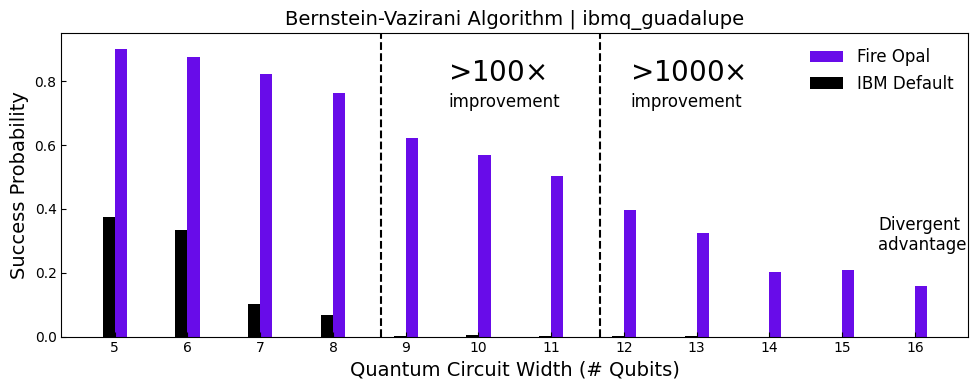With the inevitable decoherence of qubits, there are limitations to how long a system remains useful for computation. This time period is often referred to as the $T_1$ limit.

The next figure presents the success probability of the BV algorithm for the different settings, together with two projected performance bounds which assume the existence of either uncorrelated gate errors or $T_1$ processes only. The gate-error limit is calculated by a simple multiplication of the fidelities derived from the backend for all single and two-qubit gates in the circuits. The circuit $T_1$ limit is calculated by a multiplication of the $T_1$-limited fidelity of each participating qubit, calculated as $\Pi_i = \exp(-T_i / 2 T_1^{(i)})$, where $T_i$ is the time from first operation until measurement of qubit $i$.

Fire Opal extends the device quality to near $T_1$ limits enabling the execution of deeper and wider circuits, despite decline in qubit quality.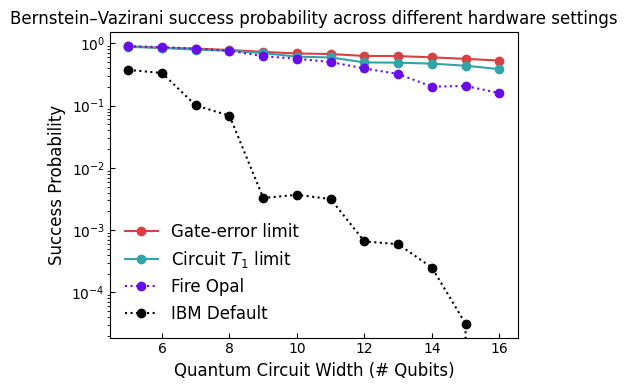The following graph shows similar effects as the previous, with circuit depth (number of 2-qubit gates) now increasing, instead of circuit width. With Fire Opal, it's possible to maintain a high solution probability, in constrast to IBM default, as the depth of the circuit increases.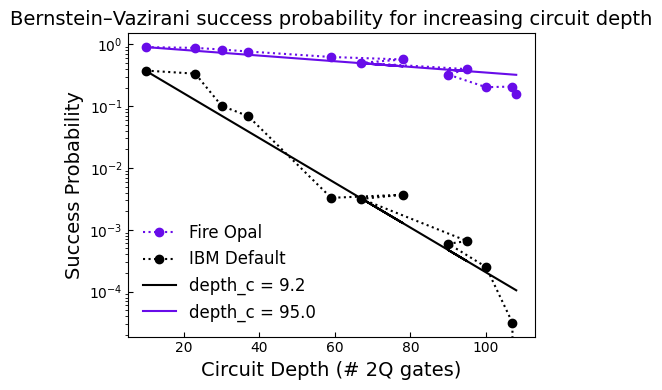### Quantum Fourier transform (QFT)

The quantum Fourier transform represents a mapping between quantum state vectors. We can efficiently estimate its performance by initializing a circuit to a state which is an inverse Fourier transform of a single bitstring. Such states can be generated with high fidelity by a single layer of Hadamard gates followed by a single layer of virtual Z rotations.

Despite the simple initialization process, these still form a full superposition of all possible bitstrings. After execution of the QFT algorithm on these initial states, the probability of finding a specific bitstring as the output of the algorithm is measured.

The circuits are usually of intermediate depth and are partially sequential. The circuit depth scales quadratically with circuit width when the underlying qubits are fully connected.

Below, the mean success probability of a QFT circuit is shown as a function of the system size, over 8 different initial states. Absolute performance is lower than that of BV, which is to be expected as QFT circuits tend to be much more complex than BV circuits. In any case, Fire Opal continues to deliver clear results as circuit width increases, while the default becomes overridden with noise.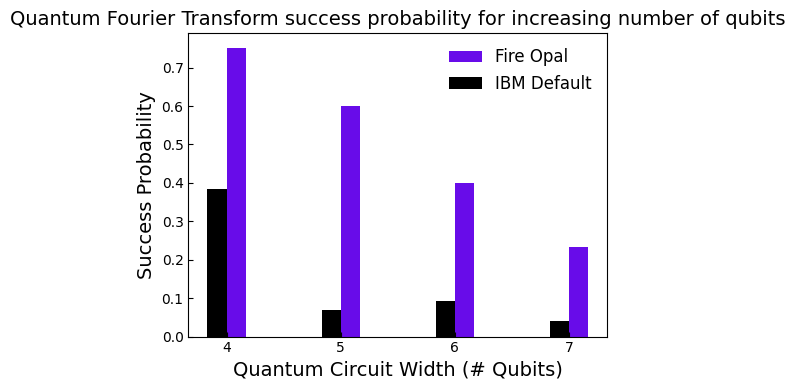The last deterministic algorithm used for benchmarking is Grover’s search, whose solution contains a predefined set of bitstrings. In this case, the solution set includes a single bitstring, and the solution of the search is encoded as the mode of the output distribution. The measured distribution over all possible bitstrings is compared to the ideal distribution in order to determine success probability.

Circuits tend to have a high depth and scaling depends on the implementation of the multi-CX gate. The depth can be reduced by allowing the addition of ancilla qubits.

In figures (a-c) below, the output probability distributions are shown over all possible 5-qubit bitstrings after two iterations of a Grover's search algorithm with 00101 as the specific oracle or solution. Figure (a) shows the ideal expected distribution calculated using a noiseless simulator, and (b-c) are probabilities obtained using default settings (black) and Fire Opal (purple). The resemblance between the measured and ideal distributions is captured by the circuit infidelity.

The distribution obtained by using Fire Opal is 8x closer to the ideal results. Similar improvement is observed for all target states. In order to quantify the usefulness of the search, the selectivity is defined as $S = \log_2(\dfrac{p_t}{p_n})$, where $p_t$ is the probability of measuring the target bitstring of the search and $p_n$ is the probability of measuring the most probable bitstring which is not the target of the search. In figure (d), selectivity is calculated for all target bitstrings, demonstrating $S > 1$ for all possible 5-qubit states using Fire Opal compared to $S < 0$ using the default settings.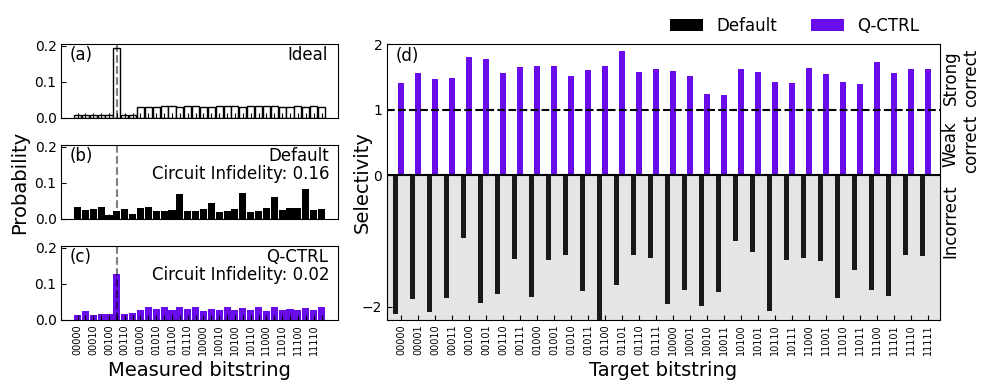### Quantum approximate optimization algorithm (QAOA)

Fire Opal now supports full closed-loop execution of QAOA algorithms in a single function call. Recent benchmarking performed demonstrated 200× improvement in reconstructing the ideal QAOA cost landscape. This new update is almost an order of magnitude better than our previous, already significant, demonstrations published in our benchmarking results and noted below. For more information on how to use Fire Opal's QAOA solver, visit our tutorial on Solving MaxCut using Fire Opal.

QAOA is the first hybrid algorithm introduced as a benchmark. It is a popular algorithm commonly used for combinatorial optimization problems with applications ranging from trasnport to finance. Hybrid algorithms are promising in the era of NISQ devices, as they enable a split of computing tasks between both a quantum and a classical processor to leverage the benefits of both.

However, this hybrid structure poses a challenge for benchmarking, since results depend on a wide range of variables outside of just the quantum processing system. In looking at results, the quantum processor performance is isolated to compare the usage of Fire Opal specifically on this optimization loop.

A typical problem Hamiltonian is chosen for QAOA in the benchmarked case, containing all single and pairwise Z terms with non-uniform coefficients, and fixing the approximation level $p$. Baseline performance is determined by calculating the cost function, which is the expectation value of the Hamiltonian, after selecting parameters using a noiseless simulator.

The same circuit is then executed on hardware, directly evaluating the output for the same range of QAOA parameters $\{\gamma, \beta\}$, and compare the measured and the ideal cost landscapes. Structural similarity index measure (SSIM) is calculated in order to quantatively compare the similarity of the cost landscapes. If QPU hardware execution were not subject to noise, the cost landscapes of generated by a simulator and a hardware execution would be identical and the SSIM would be one.

In the images below, the ideal landscape (top) is compared with the experimentally obtained landscapes using the default settings (middle) and Fire Opal (bottom).

The results show that Fire Opal significantly improves the qualitative nature of the QAOA landscape found, as well as a vast improvement of the structural similarity coefficient by 28×.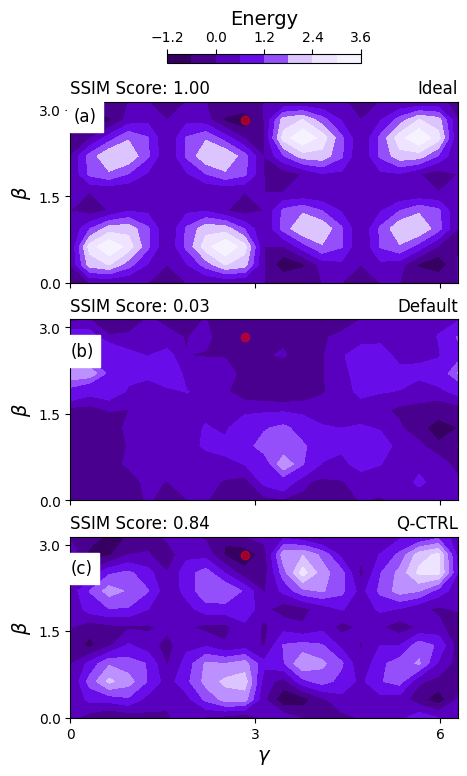### Variational quantum eigensolver (VQE)

The variational quantum eigensolver (VQE) is another hybrid algorithm that is often used in chemistry applications. VQE uses the variational principle to compute the ground state energy of a Hamiltonian by executing a parameterized circuit with a fixed form known as a variational form or an Ansatz.

The current problem under test uses 4- and 6-qubit $R_y$ ansatzes with linear entanglement. Each round of the ansatz has depth and density in the BV class.

VQE performance is benchmarked here with two different workflows. In figure (a), the ground state energy of the BeH2 molecule as a function of the Be-H bond distance is computed for a 6-qubit ansatz. The dotted line displays results from an ideal simulator, and the individual points represent the results computed on real hardware with different settings.

Figure (b) shows the deviation from expected Pauli measurement as a function of the ideal value. Perfect agreement between theory and experiment would correspond to all points on the zero dashed line.

For six qubits, the agreement between the calculated expectation values and the ideal values is 16× higher with Fire Opal compared to the default. Using Fire Opal, the deviation curve is approximately flat, which indicates that expectation values are consistently originating from the correct probability distributions.

To quantify the agreement, we calculate the Pearson distance $P_d = 1 - P_c$, where $P_c$ is the standard Pearson correlation coefficient. The Pearson distance for the default was found to be 0.177, whereas when using Fire Opal, it was found to be 0.011.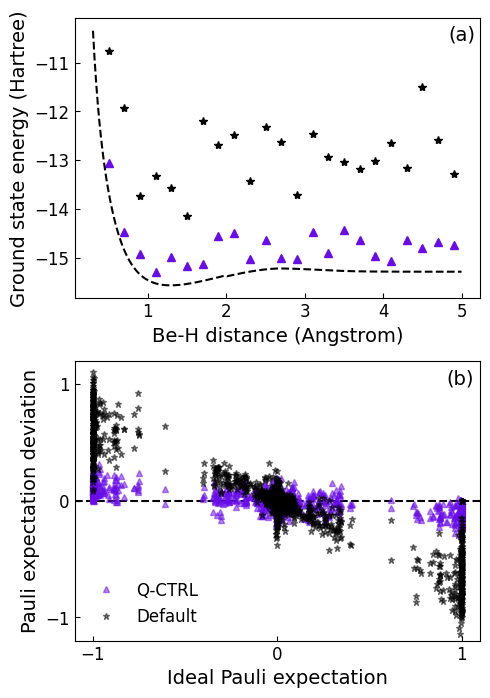### Quantum error correction (QEC) codes

Quantum error correction (QEC) is a critical task that will be required for full quantum computation. At its heart, QEC depends on an encoding, which redundantly maps $k$ logical qubits into $n > k$ physical qubits, and $n − k$ stabilizer generators, which provide partial information about hidden errors that may occur. Collectively, measurements of the stabilisers produce syndrome data, and a decoding algorithm, processes the syndrome to provide a likely correction operator that has a high probability of correcting the error.

At its core, QEC is a form of hybrid quantum-classical algorithm that generates "mid-circuit" measurement results, which is used in a classical decoding process to infer and correct for errors during the course of a logical compution. The benchmarking is based on measuring the improvement of the performance of a single iteration of the hybrid pipeline, specifically focusing on the quantum processing portion.

As a benchmarking tool, the measure of effectiveness is determined by the likelihood of identifying an error correctly.

Two error-correcting codes are tested: the 5-qubit repetition code which is capable of correcting up to two bit-flip errors, and the 5-qubit quantum error correction code, which is capable of correcting any single qubit error.

Ideally, the measured syndrome and the expected syndrome should agree, and evaluating the joint probability distribution provides a quantative way to evluate the quality of the results.

The figures below show the performance of QEC using Fire Opal compared to the Default.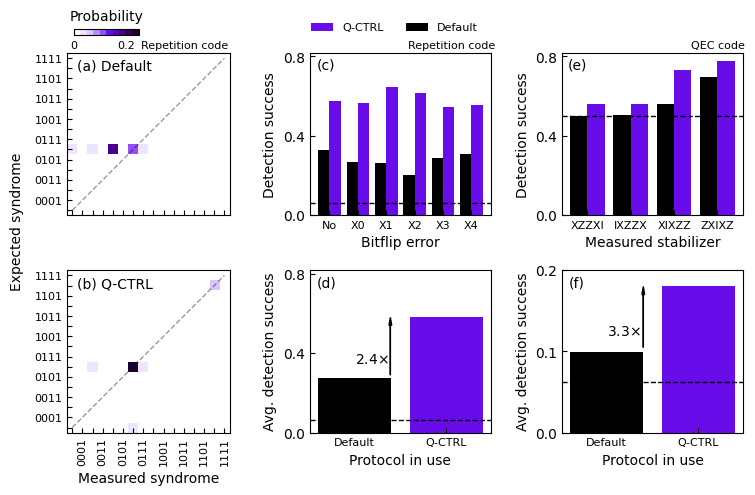Figures (a-d) show benchmarking data for the five-qubit repetition code.

Figures (a-b) show joint probability distribution of the measured and expected syndromes for the different settings after an error was injected to qubit 2. The syndrome bit values “0” or “1” correspond to the measurement outcomes “+1” and “−1” for each of the stabilizer generators.

Figure (c) shows the detection success as a function of the injected error for the default and Fire Opal using color coding consistent with the graphs shown above. The dashed line denotes the randomness threshold, which represent the success achieved by a random chance.

Figure (d) shows the mean detection success averaged over the six bit-error-location possibilities, comparing the two protocols. The arrow indicates quantitative performance enhancement, calculated with respect to the dashed line.

Figures (e-f) show data on full 5-qubit QEC.

Figure (e) shows the detection success for each of the four stabilizers measured for the different pipelines. As before, the dashed line is the random chance value.

Finally figure (f) shows the overall mean (over injected errors) detection success incorporating data averaged over all four stabilizers simultaneously with net performance enhancement with respect to the random value represented.

Results using Fire Opal are consistently higher than the default pipeline and all give an enhanced likelihood of error identification. By contrast, the two stabilizer measurements performed with default configurations are consistent with random chance. With Fire Opal, average detection success is improved by 2.5-3.3× over random chance. These results are in spite of the fact that the hardware layout used in testing is not optimized for the small demonstration codes used.